## UGC NET CS 2015 June Paper-3

 Question 1
For the 8 - bit word 00111001, the check bits stored with it would be 0111. Suppose when the wo​rd is read from memory, the check bits are calculated to be 1101. What is the data word that was read from memory?
 A 10011001 B 00011001 C 00111000 D 11000110
Digital-Logic-Design       Number-Systems
Question 1 Explanation:
Here, the check bits stored along with data are 0111 and the check bits calculated at receiver side are 1101. Both check bits are not same is the indication of error bit in data whose position can be calculated by performing the EX-OR operation.So data bits are:
00011001
 Question 2
Consider a 32 - bit microprocessor, with a 16 - bit external data bus, driven by an 8 MHz input clock. Assume that this microprocessor has a bus cycle whose minimum duration equals four input clock cycles. What is the maximum data transfer rate for this microprocessor?
 A 8 × 10​ 6​ bytes/sec B 4 × 10​ 6​ bytes/sec C 16 × 10​ 6​ bytes/sec D 4 × 10​ 9​ bytes/sec
Computer-Organization       Microprocessor
Question 2 Explanation:Question 3
The RST 7 instruction in 8085 microprocessor is equivalent to:
 A CALL 0010 H B CALL 0034 H C CALL 0038 H D CALL 003C H
Computer-Organization       Microprocessor
Question 3 Explanation: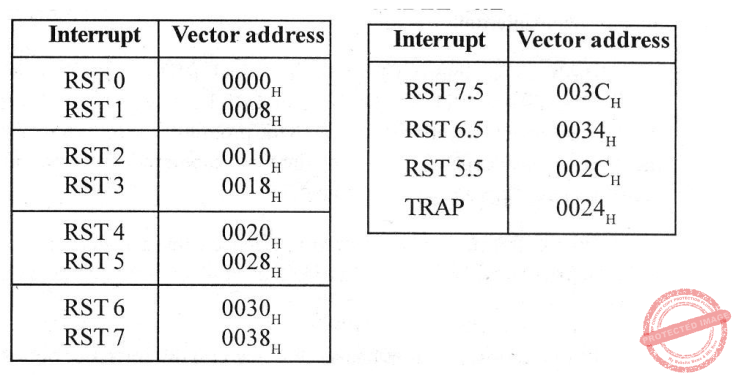Question 4
The equivalent hexadecimal notation for octal number 2550276 is:
Digital-Logic-Design       Number-Systems
Question 4 Explanation: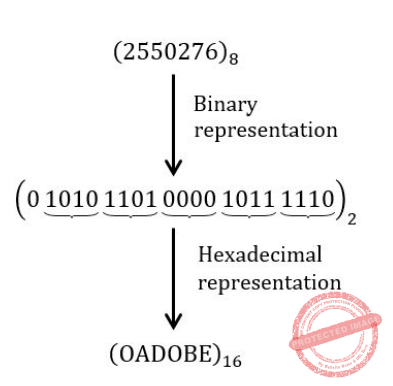Question 5
The CPU of a system having 1 MIPS execution rate needs 4 machine cycles on an average for executing an instruction. The fifty percent of the cycles use memory bus. A memory read/ write employs one machine cycle. For execution of the programs, the system utilizes 90 percent of the CPU time. For block data transfer, an IO device is attached to the system while CPU executes the background programs continuously. What is the maximum IO data transfer rate if programmed IO data transfer technique is used?
 A 500 Kbytes/sec B 2.2 Mbytes/sec C 125 Kbytes/sec D 250 Kbytes/sec
Computer-Organization       Microprogrammed-Control-Unit
Question 5 Explanation:
Given, CPU speed 10 6 instruction/sec
1 CPU instruction = 4 machine cycles
CPU utilization = 90%
Programmed IO: each byte transfer requires 4 cycles (instructions), in status, check status branch, R/W data in memory maximum data transfer rate,Question 6
The number of flip-flops required to design a modulo - 272 counter is:
 A 8 B 9 C 27 D 11
Digital-Logic-Design       Sequential-Circuits
Question 6 Explanation:
If we have “n” flip-flops then total number of combinations possible is 2​ n​ .
So total number of states possible is 2​ n​ i.e. MOD 2​ n​ .
Here 2​ n​ = 272
So n = log​ 2​ 272
n = 9
 Question 7
Let E​ 1​ and E​ 2​ be two entities in E-R diagram with simple single valued attributes. R1 and R​ 2​ are two relationships between E​ 1​ and E​ 2​ where R​ 1​ is one - many and R​ 2​ is many - many. R​ 1​ and R​ 2​ do not have any attributes of their own. How many minimum number of tables are required to represent this situation in the Relational Model?
 A 4 B 3 C 2 D 1
Database-Management-System       ER-Model
Question 7 Explanation:Question 8
The STUDENT information in a university stored in the relation STUDENT (Name, SEX, Marks, DEPT_Name) Consider the following SQL Query SELECT DEPT_Name from STUDENT where SEX = 'M' group by DEPT_Name having avg (Marks)>SELECT avg (Marks) from STUDENT. It Returns the Name of the Department for which:
 A The Average marks of Male students is more than the average marks of students in the same Department B The average marks of male students is more than the average marks of students in the University C The average marks of male students is more than the average marks of male students in the University D The average marks of students is more than the average marks of male students in the University
Database-Management-System       SQL
Question 8 Explanation:
The query is co-related( same table is used in outer and inner query) so compare each entry of Student table 1 with each entry of Student table 2.⇒ Consider this table for outer query i.e., LHS query of “>” symbol and here find avg. marks of a department because of group by constraint
i.e., avg. marks for CSE = 87.5
avg. marks for IT = 90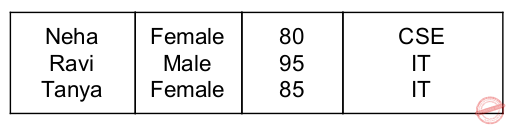⇒ Consider this table for inner query i.e., RHS query of “>” symbol and here find avg. of total marks
i.e., avg. marks = 88.7
⇓ O/P of given query is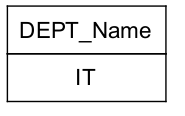Question 9
Select the 'False' statement from the following statements about Normal Forms:
 A Lossless preserving decomposition into 3NF is always possible B Lossless preserving decomposition into BCNF is always possible C Any Relation with two attributes is in BCNF D BCNF is stronger than 3NF
Database-Management-System       Normalization
Question 9 Explanation:
Option(A) is true because 3NF preserves lossless decomposition and functional dependency.
Option(B) is false because BCNF ensures lossless decomposition but do not preserves functional dependency.
Option(C) is true because Any Relation with two attributes is in BCNF
Option(D) is true because BCNF ensure less number of duplicate values in a relation than 3NF.
 Question 10
The Relation Vendor Order (V_no, V_ord_no, V_name, Qty_sup, unit_price) is in 2NF because:
 A Non_key attribute V_name is dependent on V_no which is part of composite key B Non_key attribute V_name is dependent on Qty_sup C Key attribute Qty_sup is dependent on primary_key unit price D Key attribute V_ord_no is dependent on primary_key unit price E None of the above
Database-Management-System       Normalization
Question 10 Explanation:
A relation is in 2NF if there is no partial dependency in a relation. A relation will be in 2NF if the LHS of a functional dependency is either a primary key or a non- key attribute or if all the attributes of a relation are prime attributes.
Option(A) is not correct because Non_key attribute V_name is dependent on V_no which is part of composite key i.e there is a partial dependency exists because of V_no.
Option(B) is also not correct because there are chances of having a partial dependency because Qty_sup could be a prime attribute.
Option(C) and Option(D) are correct because in both the options Key attributes depend upon primary key.
 Question 11
The relation schemas R​ 1​ and R​ 2​ form a Lossless join decomposition of R if and only if:
R​ 1​ ∩ R​ 2​ ↠ (R​ 1​ - R​ 2​ )
R​ 1​ → R​ 2
R​ 1​ ∩ R​ 2​ ↠ (R​ 2​ - R​ 1​ )
(R​ 2​ → R​ 1​ ) ∩ R​ 2
 A (a) and (b) happens B (a) and (d) happens C (a) and (c) happens D (b) and (c) happens
Database-Management-System       Functional-Dependency
Question 11 Explanation:
The decomposition of a relation is said to be lossless if and only if there exists a common attribute which is a primary key in any of the two decomposed relations. In this case statement (a) and statement (c) are satisfying this criteria. Hence option (C) is correct.
 Question 12
In the indexed scheme of blocks to a file, the maximum possible size of the file depends on:
 A The number of blocks used for index and the size of index B Size of Blocks and size of Address C Size of index D Size of Block
Operating-Systems       File system
 Question 13
Give the number of principal vanishing point(s) along with their direction for the standard perspective transformation:
 A Only one in the direction K B Two in the directions I and J C Three in the directions I, J and K D Only two in the directions J and K
Computer-Graphics       Transformations
Question 13 Explanation:
There is only one principal vanishing point and it is in K-direction.
 Question 14
Consider a triangle A(0,0), B(1,1) and C(5,2). The triangle has to be rotated by an angle of 45 about the point P(-1, -1). What shall be the coordinates of the new triangle?
 A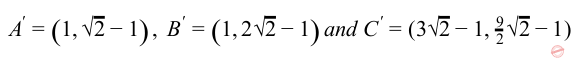BCDEngineering-Mathematics       Co-ordinate-Geometry
Question 14 Explanation: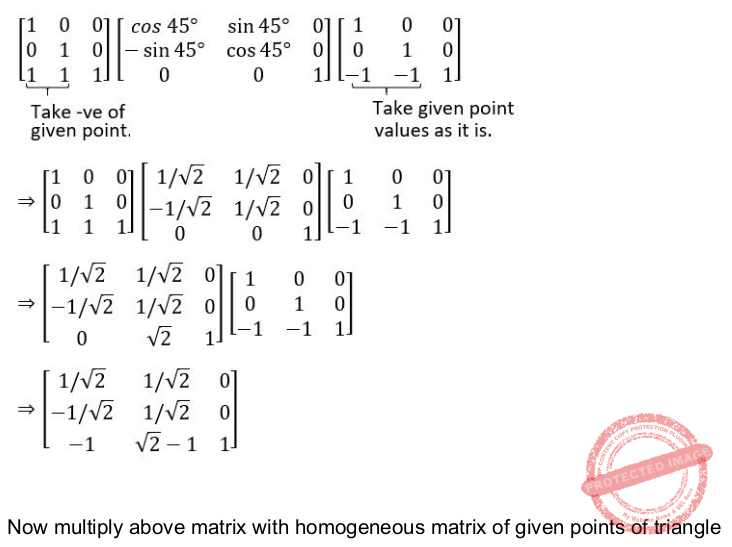Question 15
The process of dividing an analog signal into a string of discrete outputs, each of constant amplitudes, is called:
 A Strobing B Amplification C Conditioning D Quantization
Computer-Networks       Signals
Question 15 Explanation:
In analog to digital signal conversion following steps are performed: 1. Sampling: The analog signal is sampled every Ts seconds, where Ts is the sample interval or period. The result is a sequence of samples that retains the shape of the analog signal.
2. Quantization: The sampled signal is quantized. The result of sampling is a series of pulses with amplitude values between the maximum and minimum amplitudes of the signal. The set of amplitudes can be infinite with non-integral values between the two limits.
So quantization is the process of mapping a large set of input values to a smaller(countable) set.
3. Encoding: After each sample is quantized and the number of bits per sample is decided. The quantized values are encoded as streams of bits.
 Question 16
Which of the following is not a basic primitive of the Graphics Kernel System (GKS)?
 A POLYLINE B POLYDRAW C FILL AREA D POLYMARKER
Computer-Organization       Graphics
Question 16 Explanation:
Graphics Kernel System (GKS): ​ GKS provides a set of drawing features for two-dimensional vector graphics suitable for charting and similar duties. The calls are designed to be portable across different programming languages, graphics devices and hardware, so that applications written to use GKS will be readily portable to many platforms and devices.
The five main primitives in GKs are :
● Polyline: ​ which draws a sequence of connected line segments.
● Fill Area: which displays a specified area.
● Cell array: which displays an image composed of a variety of colours or grey scales.
● Polymarker: which marks a sequence of points with the same symbol.
● Text: which draws a string of characters
 Question 17
Which of the following statement(s) is/are incorrect ?
 A Mapping the coordinates of the points and lines that form the picture into the appropriate coordinates on the device or workstation is known as viewing transformation. B The coordinate system that corresponds to the device or workstation where the image is to be displayed is known as physical device coordinate system C The right-handed cartesian coordinates system in whose coordinates we describe the picture is known as world coordinate system. D Left - handed coordinate system in which the display area of the virtual display device corresponds to the unit (|x|) square whose lower left-hand corner is at the origin of the coordinate system, is known as normalized device coordinate system.
Engineering-Mathematics       Co-ordinate-Geometry
 Question 18A (a)-(i), (b)-(ii), (c)-(iii), (d)-(iv), (e)-(v) B (a)-(ii), (b)-(iii), (c)-(i), (d)-(iv), (e)-(v) C (a)-(iii), (b)-(i), (c)-(ii), (d)-(v), (e)-(iv) D (a)-(iv), (b)-(v), (c)-(i), (d)-(ii), (e)-(iii)
Computer-Graphics       Display-System
Question 18 Explanation:
Flood Gun: An electron gun designed to flood the entire screen with electrons.
Collector: Partly energized by flooding gun stores the charge generated by the writing gun.
Ground: Used to discharge the collector.
There are 18 questions to complete.

Register Now